# 高效使用Pytorch的6个技巧：为你的训练Pipeline提供强大动力

2020/09/08 10:50## 在这篇文章中，我们将介绍以下技巧：

1. 用高级框架代替自己写的循环
2. 使用另外的度量标准监控训练的进展
3. 使用TensorBoard
4. 使模型的预测可视化
5. 使用Dict作为数据集和模型的返回值
6. 检测异常和解决数值的不稳定性

# 不要重复造轮子PyTorch在从头开始编写训练循环时提供了极佳的灵活性和自由度。理论上，这为编写任何训练逻辑提供了无限可能。在实践中，你很少会为训练CycleGAN、distilling BERT或3D物体检测从头开始实现编写训练循环。

• 提供经过良好测试的训练循环
• 支持配置文件
• 支持多gpu和分布式训练
• 管理检查点/实验
• 自动记录训练进度

• 训练pipeline变得更小 —— 代码越少 —— 出错的机会就越少。
• 易于进行实验管理。
• 简化分布式和混合精度训练。

• 通常，当使用一个高级框架时，我们必须在框架特定的设计原则和范例中编写代码。
• 时间投资，学习额外的框架需要时间。

# 给我看指标Top-1准确率最好的CNN分类模型在泛化方面可能不是最好的。根据你的领域和需求，你可能希望保存具有最 false-positive/false-negative的模型，或者具有最高平均精度的模型。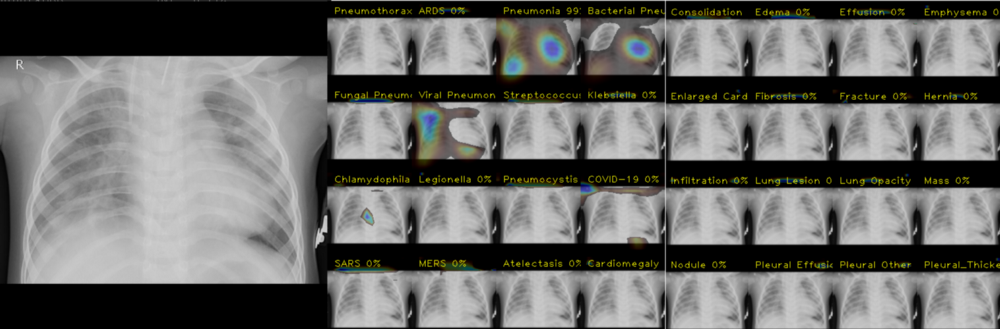• Confusion Matrix — 显示了对你的模型来说哪两个类最具挑战性。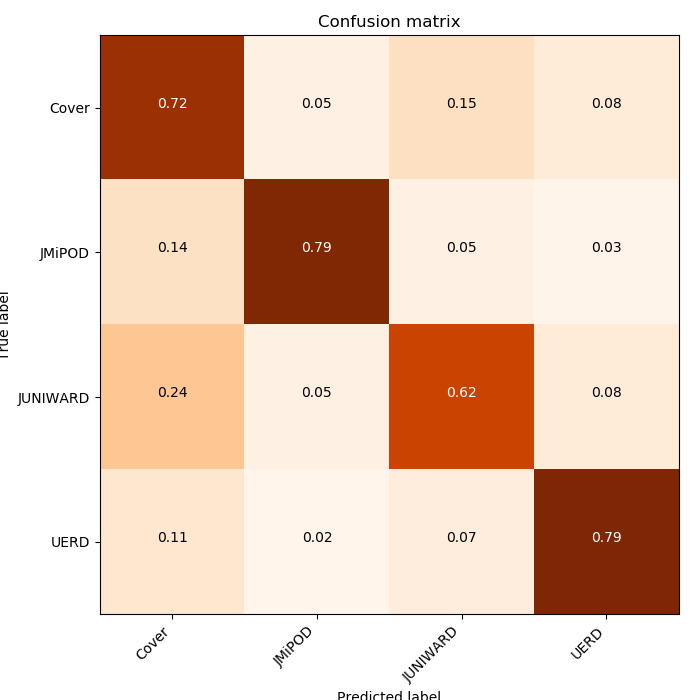• Distribution of predictions — 让你了解最优决策边界。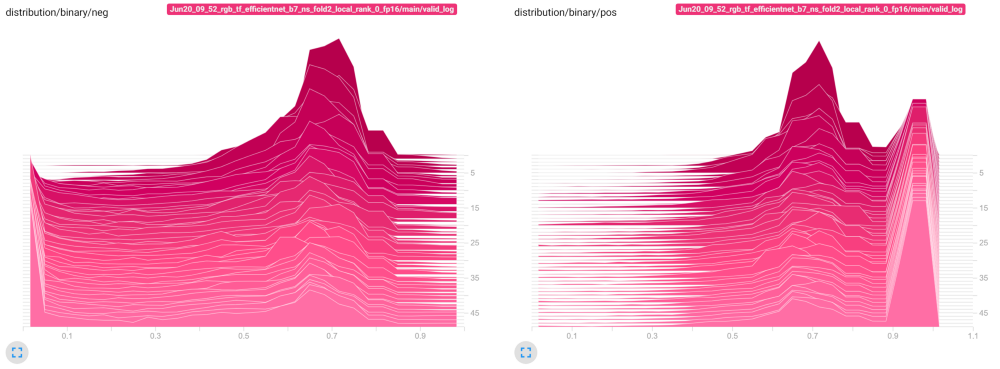• Minimum/Average/Maximum 跨所有层的梯度值，允许识别是否在模型中存在消失/爆炸的梯度或初始化不好的层。

# 使用面板工具来监控训练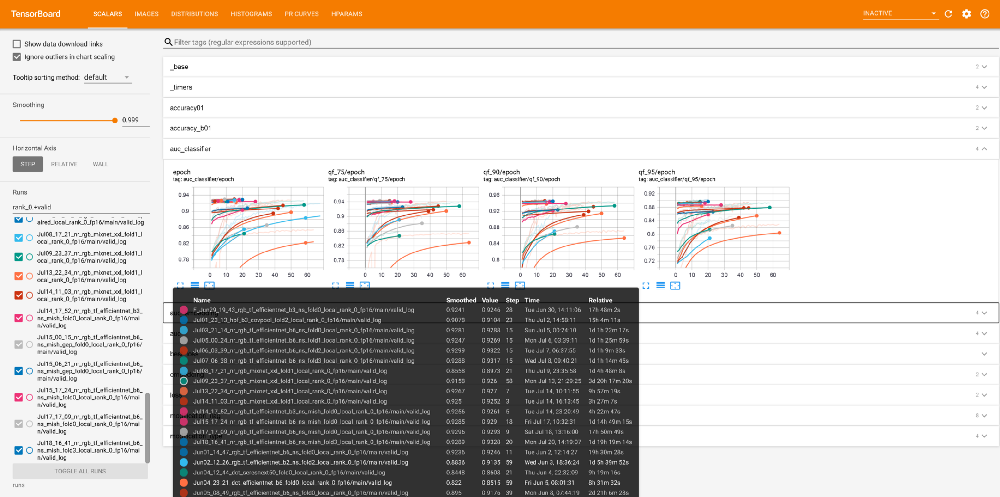Tensorboard可以快速的检查和比较你运行的训练

• 学习率和其他可能改变的优化参数(动量，重量衰减，等等)
• 用于数据预处理和模型内部的时间
• 贯穿训练和验证的损失(每个batch和每个epoch的平均值)
• 跨训练和验证的度量
• 训练session的超参数最终值
• 混淆矩阵，Precision-Recall曲线，AUC(如果适用)
• 模型预测的可视化(如适用)

# 一图胜千言

Catalyst用户提示：这里是使用可视化回调的示例：https://github.com/BloodAxe/Catalyst-Inria-Segmentation-Example/blob/master/fit_predict.py#L258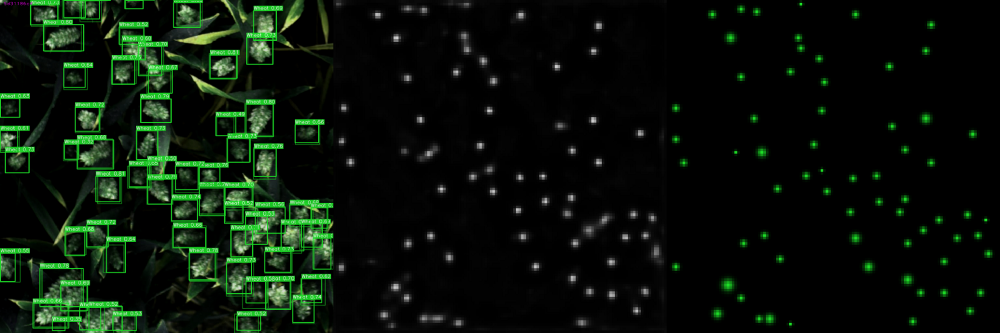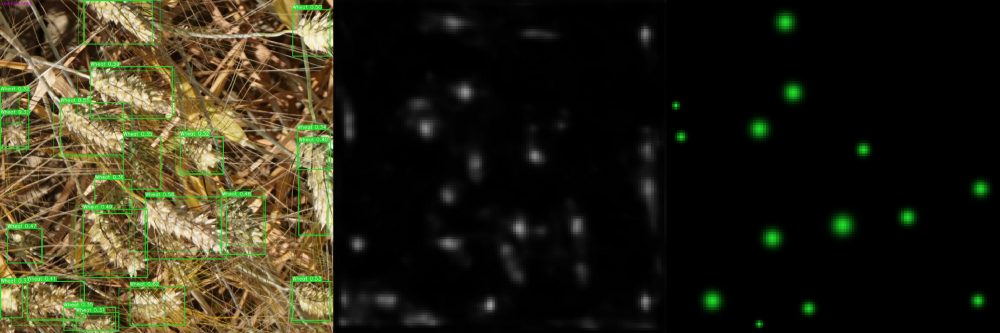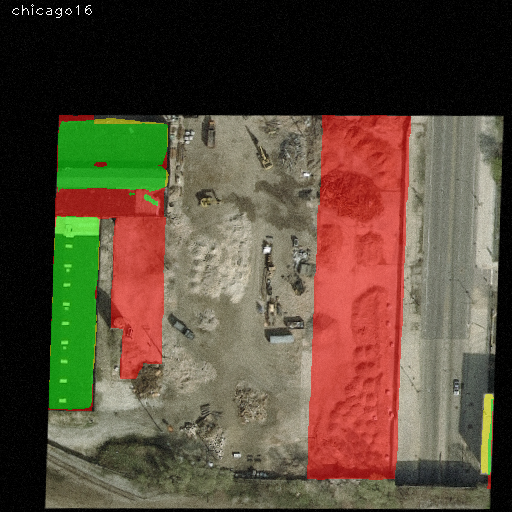# 使用Dict作为Dataset和Model的返回值

# Bad practice, don't return tupleclass RetinaNet(nn.Module):  ...  def forward(self, image):    x = self.encoder(image)    x = self.decoder(x)    bboxes, scores = self.head(x)    return bboxes, scores  ...

class RetinaNet(nn.Module):  RETINA_NET_OUTPUT_BBOXES = "bboxes"  RETINA_NET_OUTPUT_SCORES = "scores"  ...  def forward(self, image):    x = self.encoder(image)    x = self.decoder(x)    bboxes, scores = self.head(x)    return { RETINA_NET_OUTPUT_BBOXES: bboxes,              RETINA_NET_OUTPUT_SCORES: scores }  ...

• 返回值有一个显式的名称与它关联。你不需要记住元组中元素的确切顺序。
• 如果你需要访问返回的字典的一个特定元素，你可以通过它的名字来访问。
• 从模型中添加新的输出不会破坏代码。

# https://github.com/BloodAxe/Kaggle-2020-Alaska2/blob/master/alaska2/models/timm.py#L104def forward(self, **kwargs):  x = kwargs[self.input_key]  x = self.rgb_bn(x)  x = self.encoder.forward_features(x)  embedding = self.pool(x)  result = {    OUTPUT_PRED_MODIFICATION_FLAG: self.flag_classifier(self.drop(embedding)),    OUTPUT_PRED_MODIFICATION_TYPE: self.type_classifier(self.drop(embedding)),  }  if self.need_embedding:    result[OUTPUT_PRED_EMBEDDING] = embedding  if self.arc_margin is not None:    result[OUTPUT_PRED_EMBEDDING_ARC_MARGIN] = self.arc_margin(embedding)  return result

# https://github.com/BloodAxe/Kaggle-2020-Alaska2/blob/master/alaska2/dataset.py#L373class TrainingValidationDataset(Dataset):    def __init__(        self,        images: Union[List, np.ndarray],        targets: Optional[Union[List, np.ndarray]],        quality: Union[List, np.ndarray],        bits: Optional[Union[List, np.ndarray]],        transform: Union[A.Compose, A.BasicTransform],        features: List[str],    ):        """        :param obliterate - Augmentation that destroys embedding.        """        if targets is not None:            if len(images) != len(targets):                raise ValueError(f"Size of images and targets does not match: {len(images)} {len(targets)}")        self.images = images        self.targets = targets        self.transform = transform        self.features = features        self.quality = quality        self.bits = bits    def __len__(self):        return len(self.images)    def __repr__(self):        return f"TrainingValidationDataset(len={len(self)}, targets_hist={np.bincount(self.targets)}, qf={np.bincount(self.quality)}, features={self.features})"    def __getitem__(self, index):        image_fname = self.images[index]        try:            image = cv2.imread(image_fname)            if image is None:                raise FileNotFoundError(image_fname)        except Exception as e:            print("Cannot read image ", image_fname, "at index", index)            print(e)        qf = self.quality[index]        data = {}        data["image"] = image        data.update(compute_features(image, image_fname, self.features))        data = self.transform(**data)        sample = {INPUT_IMAGE_ID_KEY: os.path.basename(self.images[index]), INPUT_IMAGE_QF_KEY: int(qf)}        if self.bits is not None:            # OK            sample[INPUT_TRUE_PAYLOAD_BITS] = torch.tensor(self.bits[index], dtype=torch.float32)        if self.targets is not None:            target = int(self.targets[index])            sample[INPUT_TRUE_MODIFICATION_TYPE] = target            sample[INPUT_TRUE_MODIFICATION_FLAG] = torch.tensor([target > 0]).float()        for key, value in data.items():            if key in self.features:                sample[key] = tensor_from_rgb_image(value)        return sample

# https://github.com/BloodAxe/Kaggle-2020-Alaska2callbacks += [  CriterionCallback(    input_key=INPUT_TRUE_MODIFICATION_FLAG,    output_key=OUTPUT_PRED_MODIFICATION_FLAG,    criterion_key="bce"  ),  CriterionCallback(    input_key=INPUT_TRUE_MODIFICATION_TYPE,    output_key=OUTPUT_PRED_MODIFICATION_TYPE,    criterion_key="ce"  ),  CompetitionMetricCallback(    input_key=INPUT_TRUE_MODIFICATION_FLAG,    output_key=OUTPUT_PRED_MODIFICATION_FLAG,    prefix="auc",    output_activation=binary_logits_to_probas,    class_names=class_names,  ),  OutputDistributionCallback(      input_key=INPUT_TRUE_MODIFICATION_FLAG,      output_key=OUTPUT_PRED_MODIFICATION_FLAG,      output_activation=binary_logits_to_probas,      prefix="distribution/binary",  ),  BestMetricCheckpointCallback(    target_metric="auc",     target_metric_minimize=False,     save_n_best=3),]

# 在训练中检测异常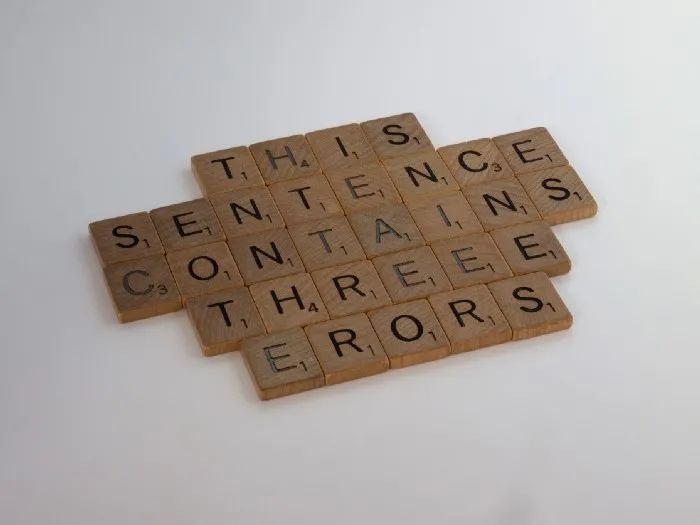• 模型或特定层的初始化不好(你可以通过观察梯度大小来检查哪些层)
• 数学上不正确的运算(负数的 torch.sqrt() ，非正数的 torch.log() ，等等)
• 不当使用 torch.mean() torch.sum() 的reduction(zero-sized张量上的均值会得到nan，大张量上的sum容易导致溢出)
• 在loss中使用 x.sigmoid() (如果你需要在loss函数中使用概率，更好的方法是 x.sigmoid().clamp(eps,1-eps )以防止梯度消失)
• 在使用fp16的训练的时候没有使用动态损失缩放

import torchdef main():    torch.autograd.detect_anomaly()    ...    # Rest of the training code   # ORclass MyNumericallyUnstableLoss(nn.Module):  def forward(self, input, target):    with torch.autograd.set_detect_anomaly(True):       loss = input * target       return loss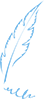END1
0 收藏

### 作者的其它热门文章0 评论
0 收藏
1